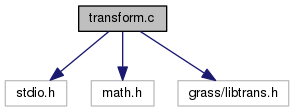GRASS Programmer's Manual  6.5.svn(2014)-r66266
transform.c File Reference

This file contains routines which perform (affine?) transformations from one coordinate system into another. More...

`#include <stdio.h>`
`#include <math.h>`
`#include <grass/libtrans.h>`
Include dependency graph for transform.c:Go to the source code of this file.

## Functions

int compute_transformation_coef (double ax[], double ay[], double bx[], double by[], int *use, int n)

int transform_a_into_b (double ax, double ay, double *bx, double *by)

int transform_b_into_a (double bx, double by, double *ax, double *ay)

int residuals_a_predicts_b (double ax[], double ay[], double bx[], double by[], int use[], int n, double residuals[], double *rms)

int residuals_b_predicts_a (double ax[], double ay[], double bx[], double by[], int use[], int n, double residuals[], double *rms)

int print_transform_matrix (void)
Prints matrix to stdout in human readable format. More...

## Detailed Description

This file contains routines which perform (affine?) transformations from one coordinate system into another.

The second system may be translated, stretched, and rotated relative to the first. The input system is system a and the output system is b.

This program is free software under the GNU General Public License (>=v2). Read the file COPYING that comes with GRASS for details.

Date
1987-2007

Definition in file transform.c.

## Function Documentation

 int compute_transformation_coef ( double ax[], double ay[], double bx[], double by[], int * use, int n )

Definition at line 90 of file transform.c.

References count, inverse(), m_mult(), and n.

 int print_transform_matrix ( void )

Prints matrix to stdout in human readable format.

Returns
int 1

Definition at line 231 of file transform.c.

 int residuals_a_predicts_b ( double ax[], double ay[], double bx[], double by[], int use[], int n, double residuals[], double * rms )

Definition at line 205 of file transform.c.

 int residuals_b_predicts_a ( double ax[], double ay[], double bx[], double by[], int use[], int n, double residuals[], double * rms )

Definition at line 214 of file transform.c.

 int transform_a_into_b ( double ax, double ay, double * bx, double * by )

Definition at line 174 of file transform.c.

 int transform_b_into_a ( double bx, double by, double * ax, double * ay )

Definition at line 183 of file transform.c.